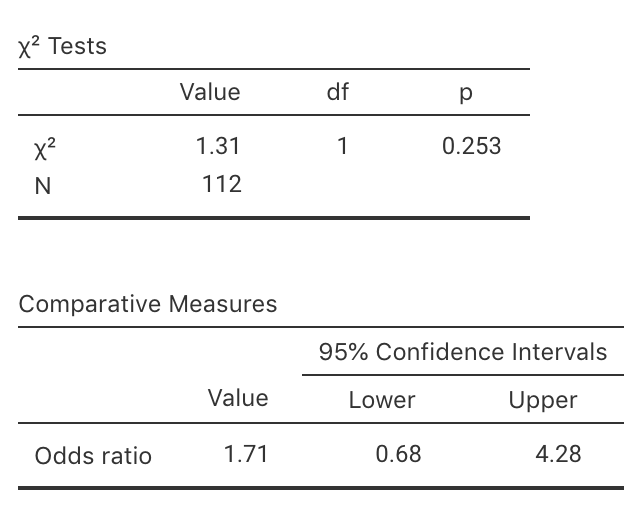## 31.12 Quick review questionsA study of the adoption of electric vehicle (EVs) by a certain group of professional Americans (Example 5.14) compiled the data in Table 31.11. Output from using jamovi is shown in Fig. 31.12.

TABLE 31.11: Responses to the question ‘Would you purchase an electric vehicle in the next 10 years?’ by education
Yes No
1. The $$\chi^2$$ value is:
2. The approximately-equivalent $$z$$-score (to two decimal places) is:
3. Using the 68–95–99.5 rule, the $$P$$-value is:
4. From the software output, the $$P$$-value is: